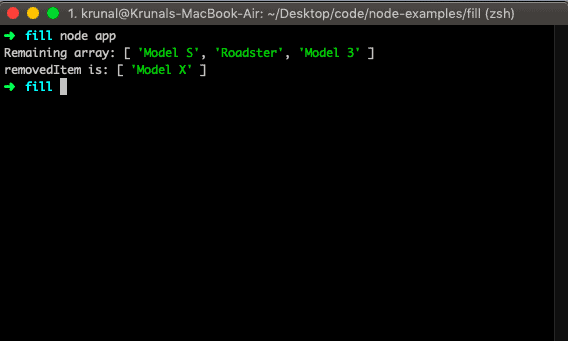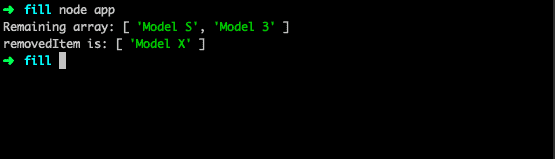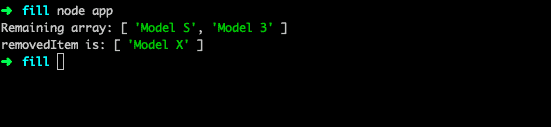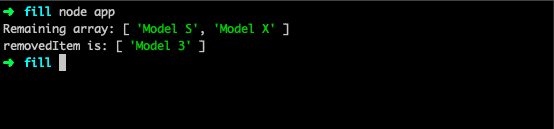# JavaScript Array Splice() Method

JavaScript array splice() is a built-in method that changes the elements of an array by removing or replacing the existing elements and/or adding new elements. The syntax is Array.splice(index, remove_count, item_list).

### Syntax

``array.splice(index, howmany, item1, ....., itemX)``

### Parameters

The first parameter is an array index, which means where to start adding or removing the item.

The second parameter is howmany means from the start index how many indexes we need to cover from the starting index.

The last parameters are optional. If we specify, it will add the items on the array by specifying the starting index.

### Example 1: How to use the array.splice() method in JavaScript

``````let tesla = ['Model S', 'Model X', 'Model 3'];
let removedItem = tesla.splice(1, 1, 'Roadster');

console.log('Remaining array:', tesla);
console.log('removedItem is:', removedItem);``````

So, here we have defined one array, and then we are changing that array by providing the first argument as a starting point of an index of 1. So it will look for index 1, which is Model X.

Now, the second parameter is also 1. So it will be the length of how many indexes we need to go to the right side of an array. So in our case, it is still Model X. The third parameter, Roadster, will replace Model X.If we do not specify the third or fourth argument, it will remove the item from an array. It will take as removing an item from the array.

### Example 2: How to get the removed element using the splice() method

``````let tesla = ['Model S', 'Model X', 'Model 3'];
let removedItem = tesla.splice(1, 1);

console.log('Remaining array:', tesla);
console.log('removedItem is:', removedItem);``````

That means Model X will be removed from an array, and we get the remaining array.

Output### Example 3: Don’t specify the second argument

Let us see the scenario where we do not also determine the second argument.

``````let tesla = ['Model S', 'Model X', 'Model 3'];
let removedItem = tesla.splice(1);

console.log('Remaining array:', tesla);
console.log('removedItem is:', removedItem);``````

If we do not specify the second argument, it will take the first one, find that index, and remove that item and other items.

So in the above example, we have given index 1, which means it will go to index 1 and remove all the elements from that index and give the remaining item. In our case, it is the Model S.### Example 4: Pass the start index negative(-)

Let us take a scenario where an array’s starting index is negative. That means it will count backward at the end of an array.

``````let tesla = ['Model S', 'Model X', 'Model 3'];
let removedItem = tesla.splice(-1);

console.log(tesla);
console.log(removedItem);``````

The above example will start backward and remove the first item from the backward point of view.

In our case, it is Model 3. So it will remove that, and if we check the Tesla array, we only have two elements in the array now.## JavaScript splice() vs slice()

The splice() method is similar to Array.prototype.slice, but unlike the slice() method, it mutates the array it is called on. It also differs because it can be used to add values to an array and remove them.

That’s it.

Javascript array indexOf()

Javascript array lastIndexOf()

Javascript array findIndex()

### 1 thought on “JavaScript Array Splice() Method”

1.thanks for the post